Introduction     Foundations     Symmetries     Factorization
Twin Primes     The Number 30     Counting Primes
Perfect Squares     Formulae     1st 1000 Primes
Magic Mirror Matrix     From Alpha to Omega     About the Author

# The Prime Spiral Sieve

## Radial Geometry and Chordal Algorithms Demystify the Prime Number Sequence"My general view of mathematics is that most of the complicated things we learn have their origins in very simple examples and phenomena." – Dr. Richard Evan Schwartz, Chancellor's Professor of Mathematics, Brown University

"Deep and satisfying mathematics can arise from simple beginnings." – Vicky Neale, PhD, Additive Number Theory

"Everything should be made as simple as possible, but not simpler." – Albert Einstein

"Simplicity is the ultimate sophistication." – Leonardo Da Vinci

"Seek simplicity and distrust it." – Alfred North WhiteheadThis work is licensed by its author, Gary William Croft, under a Creative Commons Attribution-ShareAlike 3.0 Unported License

### Introduction

Prime numbers have been deemed mysterious for more than three millennia. Any claim to have demystified them, as the title of this work boldly declares, will justifiably be met with skepticism. Regardless, deciphering how primes distribute in literal, mathematical terms deepens the mystery when you consider the profoundly beautiful algorithms and rotating symmetry groups that govern where on the number line these atoms of the number universe fall.

We explore these patterns in great depth with a powerful analytical tool: a deterministic algorithm and ever-expanding geometry in the form of a spiral sieve (the 'Prime Spiral Sieve'). It's Archimedean orbits encompass eight arithmetic progressions that double as multiplicative initiators of geometrically expanding period-24 factorization cycles. These deterministic sequences intertwine like an octal helix and ultimately determine the distribution of all prime numbers greater than 5, i.e., starting with 7.

We will also demonstrate how the Prime Spiral Sieve can geometrically shapeshift into a rectangular matrix, 4-sided pyramid, or continuous triangular wave.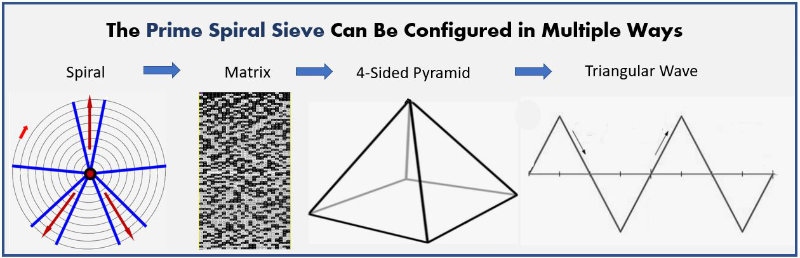And we will show how the first three primes, 2, 3, and 5–although excluded from our factorization domain by definition–are nonetheless omnipresent in the form of structure serving to contain and direct the aforementioned factorization algorithms.

One might call what follows 'primal math' in that its modular arithmetic is revealed to be deeply rooted in the most deceptively primitive mathematics of all–digit sum and digital root arithmetic (and especially the Vedic Square that originated in ancient India). This methodology combining congruence relations with digital root cycles exposes palindromes and complex polygons that illuminate our understanding of how prime numbers are formed.

The patterns in question are revealed in hybrid period-24 digital root ↔ modulo 90 congruence matrices found in our section devoted to Factorization and in a paper by the undersigned titled "From Vedic Square to the Digital Root Clockworks of Modulo 90 Factorization" published in the September, 2018 edition of the online International Journal of Vedic Mathematics (Note: link opens .pdf).

To date this site has attracted more than 106,157 "absolute unique visitors" (Google Analytics speak) from 11,124 cities and towns representing every country on Earth. Scores have returned repeatedly, and not one has offered counter-examples. Commenting on LinkedIn.com, a NASA data scientist said of this work: It "is truly a well thought out labor of mathematical thought." From a hermeneutic perspective, the patterns explored herein speak eloquently for themselves ... Res ipsa loquitur ...

Eighteen of the sequences discussed on this site have been published by the author on the On-Line Encyclopedia of Integer Sequences. Here's a link to them: OEIS Listings for Gary W. Croft

Also, an M.I.T. licensed (Python) Prime Factorization Tool compared seven non-probabilistic (aka deterministic) prime number sieving algorithms, and the programmer deemed the Croft Spiral (aka Prime Spiral Sieve) the 'fastest and most efficient' of those tested. Quoting the programmer, "The fastest method, Croft, is over 1000 times faster than the slowest."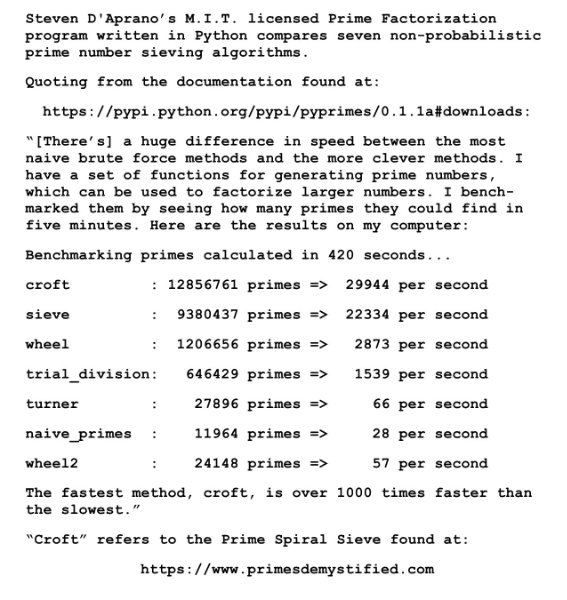The 'Croft Spiral Sieve' algorithm is listed under Python at Rosetta Code's site devoted to "Prime Decomposition" and can also be found posted on the University of Massachusetts Boston, College of Science and Mathematics (Department of Computer Science) server, via this link (which opens a .pdf): https://www.cs.umb.edu/~eb/480/factoring/croft.pdf.

Further validation comes from Fredrick Michael, PhD (Director Experfy Institute / Harvard Innovation Laboratories), who utilized this site's period-24 model of primes and composites in a study of statistics and modular arithmetic of prime numbers. An abstract of the referenced study can be found at Notes on Prime Numbers, Their Numerical Statistics & Patterns I: Modular Arithmetic and the Eight Fold Period 24 Model. The period-24 patterns in question, relating to modulo 90 factorization algorithms and the digital root dyad cycles generated by twin primes, are discussed at length on our page devoted to them: Factorization and prime number sieving algorithms and geometries..

The sequence populating the Prime Spiral Sieve (pictured below) can be variously defined as:

♦  Natural numbers coprime to 30, i.e., not divisible by 2, 3 or 5. And given no prime number > 5 is divisible by 2, 3 or 5, it's axiomatic that our factorization domain contains all prime numbers > 5, starting with 7 (the number 1 being "silent")... and their multiplicative multiples, beginning with 7 x 7 = 49, the first composite number in the sequence. It follows that all members of our domain are relatively prime (aka coprime or mutually prime) to 2, 3 and 5.

♦  Natural numbers ≌ {1, 7, 11, 13, 17, 19, 23, 29} modulo 30, which parse into 8 arithmetic progressions, each with a common difference of 30 between consecutive terms.

♦  Group U(30) = {1, 7, 11, 13, 17, 19, 23, 29} of positive integers smaller than and relatively prime to 30 with multiplication modulo 30.

♦  1 {+6 + 4 + 2 + 4 + 2 + 4 + 6 + 2} {repeat ... ∞}.

♦  30n+1, 30n+7, 30n+11, 30n+13, 30n+17, 30n+19, 30n+23, 30n+29.

♦  Natural numbers modulo 30 that distribute to the following 8 angles:

12° ... 84° ... 132° ... 156° ... 204° ... 228° ... 276° ... 348°.

♦  All odd numbers with digital root of 1, 2, 4, 5, 7 or 8 and final digit of 1, 3, 7 or 9, the first 8 of which are 1, 7, 11, 13, 17, 19, 23, 29.

[Note: Throughout this site you'll find references to various cumulative sum and indexing formulae. For convenience, we've created a page dedicated to them: Cumulative Sum and Indexing Formulae.]

It's important to emphasize that the beautiful symmetries encountered within this factorization domain, both numeric and geometric, are largely a consequence of patterns rooted in its period-24 digital root, which has the following repetition cycle:

{1, 7, 2, 4, 8, 1, 5, 2, 4, 1, 5, 7, 2, 4, 8, 5, 7, 4, 8, 1, 5, 7, 2, 8} {repeat ...}.

This 24-beat cycle is equivalent to three rotations around the Prime Spiral Sieve. The first 24 members of our domain and their digital roots, therefore, are especially important given they set the initial conditions for all that follows. To emphasize this period-8 / mod 30 telescopic stretch to period-24 / modulo 90, we occasionally group this sequence as:

n ≌ {1, 7, 11, 13, 17, 19, 23, 29, 31, 37, 41, 43, 47, 49, 53, 59, 61, 67, 71, 73, 77, 79, 83, 89} modulo 90.

The modulo 90, period-24 digital root repetition cycle of our domain allows us to sieve and sequence by digital root (dr), as listed below (and documented by the author on the On-Line Encyclopedia of Integer Sequences):

dr 1 = n ≌ {1, 19, 37, 73} mod 90: 1{+18+18+36+18} {repeat ...} (oeis.org/A301617)
dr 2 = n ≌ {11, 29, 47, 83} mod 90: 11{+18+18+36+18} {repeat ...} (oeis.org/A301621)
dr 4 = n ≌ {13, 31, 49, 67} mod 90: 13{+18+18+18+36} {repeat ...} (oeis.org/A301622)
dr 5 = n ≌ {23, 41, 59, 77} mod 90: 23{+18+18+18+36} {repeat ...} (oeis.org/A301623)
dr 7 = n ≌ {7, 43, 61, 79} mod 90: 7{+36+18+18+18} {repeat ...} (oeis.org/A301628)
dr 8 = n ≌ {17, 53, 71, 89} mod 90: 17{+36+18+18+18} {repeat ...} (oeis.org/A295869)

When the modulo 90 matrix listed above is color-coded to highlight its 12 lateral 90-sums (12 x 90 = 1080 = 360 x 3), perfect symmetry is exposed: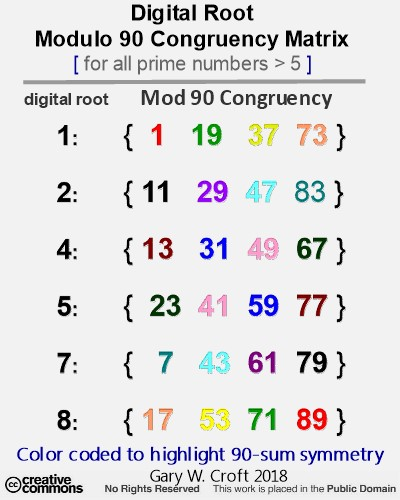In our section devoted to twin primes we demonstrate how to configure the above table to sieve and/or sequence twin prime candidates by digital root dyads.

[Note: The easiest and fastest way to determine modulo 90 congruence of a whole number is to reduce all digits antecedant to the number's terminating digit to their digital root. For example, the 1st three digits of 2497 reduce to digital root 6 leaving 67 which equates to 2497 ≌ {67} mod 90. Keep in mind that 9's count as zero when calculating digital root. This is sometimes called 'casting out 9's.']

Below is a picture of the Prime Spiral Sieve showing rotations spanning numbers 1 thru 259 of the infinite sequence populating the sieve, as defined above: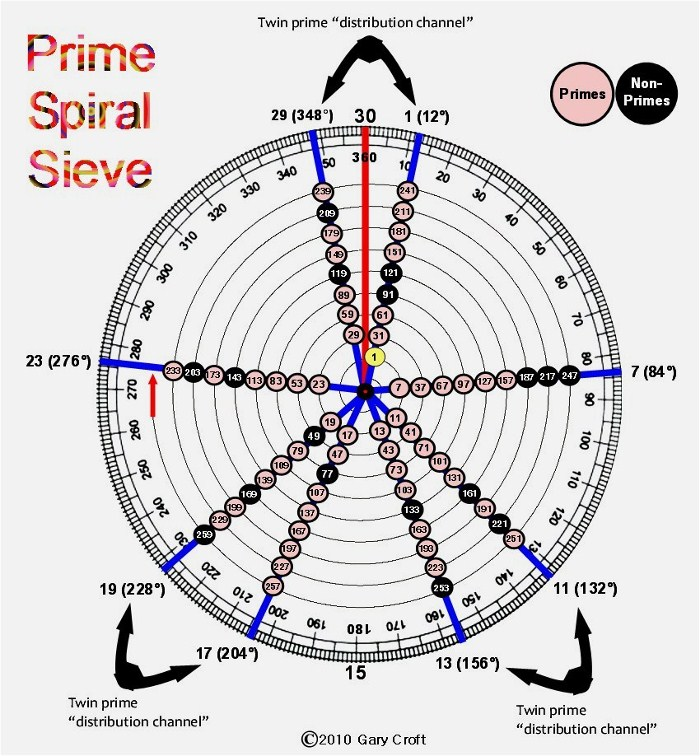Hidden deep within this sieve, a radical spin on modulo 30 wheel factorization, are mysteriously beautiful symmetries and geometries, profound in their implications. This sieve not only forms the basis of an extremely efficient and widely used prime number sieving algorithm of the 'non-probabilistic' kind (which we provided links to, above), but it demonstrates how prime numbers are distributed within a radial geometry that effectively defragments the Ulam Spiral and ultimately leads us to the Theory of Everything.

This domain, once fathomed, reveals itself to be a beautiful mathematical object. It can be conceived as both an infinite spiral and–when matrix factorized at the digital root level–an ever-expanding 4-sided pyramid structured by palindromic sequences. Regardless, the real power of this geometry becomes evident when we triple it dimensionally and explore modulo 90 factorization symmetries at the digital root level, culminating in the Magic Mirror Matrix, a calculatory geometry that serves as a prime factorization sequencer accounting for the first 1000 prime numbers, exactly, and ultimately all prime numbers > 5.

Most profoundly, we'll discover how the eight spiraling factorization algorithms, i.e., the multiplicative multiples of the domain's sequence starting with 72 that ultimately account for every composite number within the Prime Spiral Sieve's orbits, are structured by rotational symmetry groups in the shape of equilateral triangles ( {1,4,7} {2,5,8} {3,6,9} ). We'll demonstrate how these can be configured to form 3 x 3 matrices which in turn extrapolate into beautiful complex polygons.

Only elementary arithmetic and geometry are required to understand what you find on this site. This minimal requirement is reflected by Mazur and Stein in Prime Numbers and the Riemann Hypothesis: "It is striking how little you actually have to know in order to appreciate the revelations offered by numerical exploration."

### Foundations

"[S]urprisingly, playing with arithmetic can lead us to unexpected and profound discoveries that point toward deeper mathematics and sometimes even deeper science." – Pradeep Mutalik, PhD., "The Puzzling Power of Simple Arithmetic," Quanta, April 20, 2021.

The genesis of most if not all repeating prime number patterns described in the mathematics literature, e.g., Twin Primes, Cullen Primes, Chen Primes, Sexy Primes, Cousin Primes, Sophie Germain Primes, Siamese Primes, Cunningham Chains ... the list goes on and on ... can be readily deciphered using the Prime Spiral Sieve as an analytical tool employing modular arithmetic (and specifically, modulo 30 relationships). Here are two examples supporting this claim, i.e. using this sieve to analyze and predict Siamese Primes (n2 -2 and n2 +2 are primes) and Sophie Germain Primes (p and 2p+1 are primes), keeping in mind that these will make more sense after you've read what follows.

The most obvious of these repeating prime patterns are the three Twin Prime Distribution Channels, described at length on this site. These and all other such repeating–albeit intermittent and seemingly random–patterns are fundamentally sub-patterns of the set of natural numbers not divisible by 2, 3 or 5 when arrayed in 8 dimensions, whether in a matrix or spiral form. The illusion of randomness results from the overlapping sequences of the eight algorithmic "chord progressions" that factorize the domain. We will be discussing these progressions when we get to prime factorization.

The first rotation of the sieve, comprised of 8 members, (1, 7, 11, 13, 17, 19, 23 and 29), is the deterministic key to everything that follows. These are the first 8 counting numbers not divisible by 2, 3 or 5, a sequence which by definition includes (and only includes) 1 and all primes ≥ 7 and their multiplicative multiples (and, as you'll see below, it's conjectured that the entire set can be generated by a simple expression involving 2, 3 and 5). The inference of this conjecture is that all prime numbers greater than 5, i.e., starting with 7, can be produced by this expression.

[Note: Given our domain is limited to numbers ≌ {1,7,11,13,17,19,23,29} modulo 30, only ϕ(m)/m = 8/30 or 26.66% of natural numbers N = {0, 1, 2, 3, ...} need be sieved. For example, to illustrate the proportionality of this ratio, we find that 25% of the first 100 natural numbers are prime, while 72% of numbers not divisible by 2, 3, or 5 are prime (and, curiously, if we count 2, 3, and 5 in after the fact, we get 75%, or exactly 3 x 25%). Also note that if you plug the number 30 into Euler's totient function, phi(n): phi(30)= 8, with the 8 integers (known as totatives) smaller than and having no factors in common with 30 being: 1, 7, 11, 13, 17, 19, 23 and 29, i.e., what are called "prime roots" above. Thirty is the largest integer with this property.]

The integer 30, product of the first three prime numbers (2, 3 and 5), and thus a primorial, plays a powerful role organizing the array's perfect symmetry, viz., in the case of the 8 prime roots:

1+29=30; 7+23=30; 11+19=30; and 13+17=30.

In The Number Mysteries well-known mathematician Marcus Du Sautoy writes: "In the world of mathematics, the numbers 2, 3, and 5 are like hydrogen, helium, and lithium. That's what makes them the most important numbers in mathematics." Although 2, 3 and 5 are the only prime numbers not included in the domain under discussion, they are nonetheless integral to it: First of all, they sieve out roughly 73% of all natural numbers, leaving only those nominally necessary to construct a geometry within which prime numbers can be optimally arrayed. The remaining 26.66% (to be a bit more precise) constituting the array can be constructed with an elegantly simple interchangeable expression (or power series, if you prefer) that incorporates the first three primes. It's conjectured that this manifold series ultimately consists of all (and only) the numbers not divisible by 2, 3, or 5 (and their negatives), which inclues all prime numbers >5 (more below under the heading "Conjectures and Facts Relating to the Prime Spiral Sieve").

What is critical to understand, is that the invisible hand of 2, 3 and 5, and their factorial 30, create the structure within which the balance of the prime numbers, i.e., all those greater than 5, are arrayed algorithmically–as we shall demonstrate. Primes 2, 3 and 5 play out in modulo 30-60-90 cycles (decomposing to {3,6,9} sequencing at the digital root level). Once the role of 2, 3 and 5 is properly understood, all else falls beautifully into place.

### Deep Symmetries

"Physicists have exalted symmetry to the position of the central concept in their attempts to organize and explain an otherwise bewildering and complex universe." Mario Livio, The Equation that Couldn't Be Solved: How Mathematical Genius Discovered the Language of Symmetry

The Prime Spiral Sieve possesses remarkable structural and numeric symmetries. For starters, the intervals between the prime roots (and every subsequent row or rotation of the sieve) are perfectly balanced, with a period 8 difference sequence of: {6, 4, 2, 4, 2, 4, 6, 2}. The entire domain can thus be defined as 1 {+6 +4 +2 +4 +2 +4 +6 +2} {repeat ... ∞}. As we've already suggested, the number 30 figures large in our modulo 30 domain. The Prime Spiral Sieve is Archimedean in that the separation distance between turns equals 30, ad infinitum. The first two rotations increment as follows:Interestingly, the sum of the 2nd rotation = 360, the product of the first three primorials, 2 x 6 x 30 = 360, and when you multiply the first five Fibonacci numbers in sequence, you produce 1, 2, 6 and 30? And, speaking of the Fibonacci number sequence, there is symmetry mirroring the above in the relationship between the terminating digits of Fibonacci numbers and their index numbers equating to members of the array populating the Prime Spiral Sieve: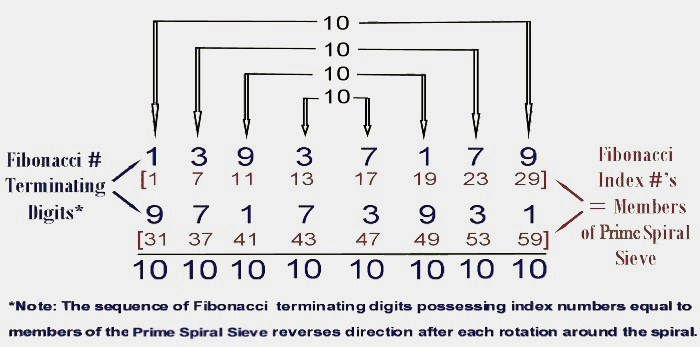Remarkably, the sequence of Fibonacci terminating digits indexed to our domain (natural numbers not divisible by 2, 3 or 5), 13,937,179 (see graphic, above), is a prime number and a member of a twin prime pair (with 13,937,177). In case you're wondering, 13,937,179 is not a reversible prime (as the reversal is a semi-prime: 9,461 x 10,271 = 97,173,931). However, given all the repunits that follow, we take note that both of the reversal's factors are congruent to 11 (mod 30 & 90). [Note: Repunits are abbreviated Rn, where n designates the number of unit 1's. Thus 1 is R1 and 11 is R2.]

Perhaps most remarkable of all, 13,937,179 when added to its reversal 97,173,931 = 111,111,110 (in strict digital root terms, the sum is 11,111,111, or R8) and the entire repeating (and palindromic) Fibo sequence end-to-end (equivalent to two rotations around the sieve) gives you this palindromic equivalency: 1,393,717,997,173,931 ≌ 11,111,111 (mod 111,111,110)... (and interestingly, 11,111,111 * 111,111,110 = 123456776543210).

Another point of interest: the terminating digits of the first 8 Fibonacci numbers indexed to our domain (13937179) contain two each 1's, 3's, 7's, and 9's. This is also true of the terminating digits of the first eight members of our domain (17137939).

Echoing the Fibonacci patterns just described, the terminating digits of the prime roots (17,137,939), when added to their reversal (93,973,171) = 111,111,110. [And note that 111,111,111 * 111,111,110 = 12345678876543210.].

Yet another related dimension of symmetry: The terminating digits of the prime root angles (24,264,868; see illustration of Prime Spiral Sieve) when added to their reversal (86,846,242) = 111,111,110, not to mention this sequence possesses symmetries that dovetail perfectly with the prime root and Fibo sequences.

And when you combine the terminating digit symmetries described above, capturing three rotations around the sieve in their actual sequences, you produce the ultimate combinatorial symmetry: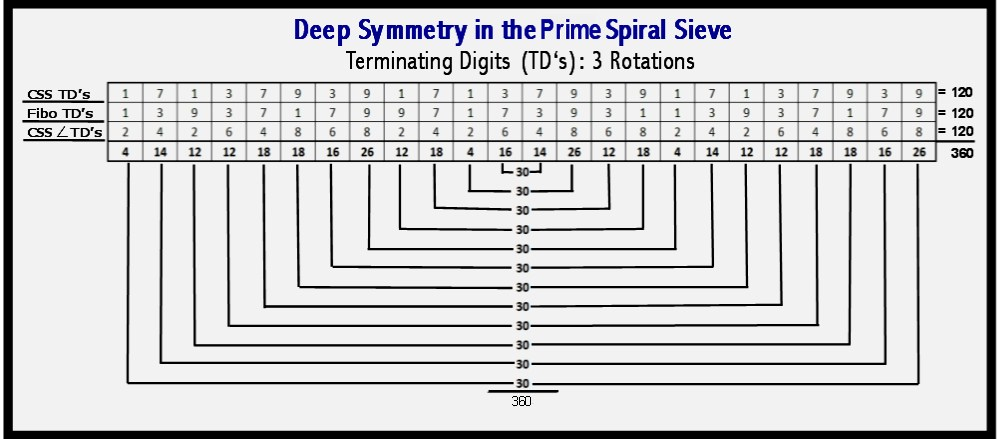Here's yet another fascinating dimension of symmetry: the pattern of 9's created by decomposing and summing either the digits of Fibonacci numbers indexed to the first two rotations of the spiral (a palindromic pattern {1393717997173931} that repeats every 16 Fibo index numbers) or, similarly, decomposing and summing the prime root angles. The decomposition works as follows (in digit sum arithmetic this would be termed summing to the digital root): F17 (the 17th Fibonacci number) = 1597 = 1 + 5 + 9 + 7 = 22 = 2 + 2 = 4:Another dimension of symmetry involves the terminating digits of the prime roots and their angles: those paired with like terminating digits being separated by 120°: 1(12°) and 11(132°) ... 13(156°) and 23(276°) ... 7(84°) and 17(204°) ... 19(228°) and 29(348°). Another consideration with regard to terminating digits, is that one can easily construct, by combining all numbers with the same terminating digits, a four-fold arithmetic progression in increments of +10 and +20, starting with 1, 7, 13 and 19. Thus, combining 1(12°) and 11(132°) gives us: 1, 11, 31, 41, 61, 71, 91, [+10+20] ... n; combining 7(84°) and 17(204°) gives us 7, 17, 37, 47, 67, 77, 97, [+10+20] ... n; combining 13(156°) and 23(276°) gives us 13, 23, 43, 53, 73, 83, 103, [+10+20] ... n; and, combining 19(228°) and 29(348°) gives us 19, 29, 49, 59, 79, 89, 109, [+10+20] ...n. Looking at the array in this configuration, however, has borne no fruit.

As fascinating as the symmetries examined above may be, they are but a prelude to the beautiful patterns we'll explore when we discuss digital root sequencing and the Trinity of Triangles and Magic Squares rooted in Vedic Arithmetic that drive factorization algorithms within this domain. And, finally, if you want to jump ahead and view the perfect palindromic symmetry found at the heart of factorization, check out the Magic Mirror Matrix that maps factorizatons at the digital root level and accounts for the first 1000 prime numbers–exactly.

### The Prime Spiral Sieve

Around the perimeter of the spiral sieve (pictured below) you'll note that the 8 radii are labeled in relation to their modulo 30 prime roots, i.e., 1(12°); 7(84°); 11(132°); 13(156°); 17(204°); 19(228°); 23(276°) and 29(348°). These relate to the fact that the circle is segmented into 30 equal sectors or radii separated by 12° (30*12°=360°), although only the eight radials that are the focus of this study are shown.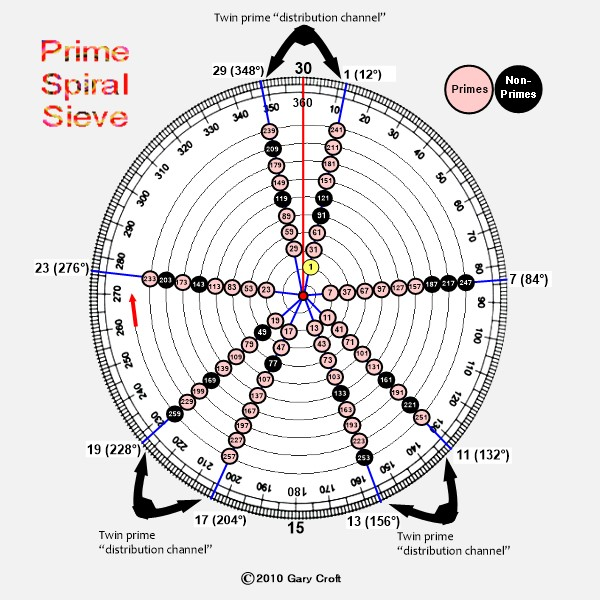This sieve exposes the twin primes, aligning as they do along three distinct distribution channels. One obvious implication, is that those numbers in the array congruent to {7} modulo 30 (radial angle 84°) and {23} modulo 30 (radial angle 276°) can be excluded as twin prime candidates (and, by definition, all prime numbers distributed along these two diagonals. with the exception of 7, which is twinned with 5, will be what are known as "isolated primes"). Later we explain how twin prime candidates can be segregated from all other positive integers and be partitioned into three columnar sets covertly aligned by the first three prime numbers (encoded in angles).

### Conjectures and Facts Relating to the Prime Spiral Sieve

The array is rooted in the first three prime numbers: 2, 3 and 5 and their product, 30, the 3rd primorial. This array reveals that the first three primes play a very special role in creating the symmetrical geometries that align the distribution of all subsequent prime numbers, thus distinguishing them from all other primes. Primes 2, 3 and 5 are like 8-legged spiders assigned to spin the beautiful spiraling web in which the remaining prime numbers are arrayed along assigned threads. (For a detailed listing of Number 30's attributes, plus reference links click here: The Number 30).

It is conjectured that all (and only) the numbers in this array (and their negatives) can be derived using the interchangeable expression incorporating the first three prime numbers, 2, 3 and 5, where x=2, y=3 and z=5. Thus: xnyn ± zn, xnzn ± yn and ynzn ± xn. For example: 2 * 3 + 5 = 11 ... 23 * 5 - 33 = 13 ... 32 * 5 + 2 = 47 ... 52 * 3 - 2 = 73. This expression, therefore, potentially generates all numbers not divisible by its three terms, 2, 3 and 5, including all prime numbers >5. [Note: For any given number in the array, there are multiple–and possibly an infinite number of–solutions. For example, the number 11 can be expressed as xy+z = 11, x2y2-z2 = 11, z2y-x6 = 11, etc.]. More examples, below:All prime numbers (with the exception of 2, 3 and 5) are distributed along 8 diagonals in intervals of 30, starting with "prime roots": 1, 7, 11, 13, 17, 19, 23 and 29 (thus: 1...31...61...91...n; 7...37...67...97...n; etc.).

The products of any combination of factors in the array = a number in the array, e.g., 7*11 = 77; 7*11*13 = 1001; etc. Conversely, all factors for composite numbers in the array can be found in the array.

Every composite number in our modulo 30 domain can be derived from the product of two terms in the domain multiplied together, and these multipliers need not necessarily be prime themselves. For example, 5831, which is congruent to 11, modulo 30, and therefore in the array, is the product of 49 x 119 = 5831. In this example, neither 49 (7 x 7) nor 119 (7 x 17) are prime, though both are members of the array.

The sum of any sequential odd number of addends in the array = a number in the array, e.g., 1+7+11 = 19; 1+7+11+13+17 = 49; etc.

Because the digital roots of all prime root angles are either 3 or 6, any prime root angle times another will produce a product whose digital root = 9, e.g., PR7 (84°) x PR29 (348°) = 84 x 348 = 29232 = dr(9).

Any number in the array x 30 + 1 = a number in the array.

The sum of the angles for 2(24°), 3(36°) and 5(60°) = 120°, and the sum of the prime roots (1+7+11+13+17+19+23+29) also = 120. This is because the prime roots are an arithmetic anagram for the angles of the first three primes, thus: 11+13 = 24; 17+19 = 36; and 1+7+23+29 = 60. The sum of the second rotation = 360 ... 3(2[24°] + 3[36°] + 5[60°]) = 30[360°]

The array reveals beautifully symmetrical relationships:

1[12°] + 29[348°] = 30[360°]
7[84°] + 23[276°] = 30[360°]
11[132°] + 19[228°] = 30[360°]
13[156°] + 17[204°] = 30[360°]

Mod 30 of all numbers in this array (and thus all primes other than 2, 3 and 5) must be 1, 7, 11, 13, 17, 19, 23 or 29.

The sum of the digital root sums of the prime roots (1, 7, 11, 13, 17, 19, 23, 29) = 1+7+2+4+8+1+5+2 = 30.

This sieve reveals why all primes >5 are adjacent to a multiple of six, as the prime root radii are adjacent to 6(72°); 12(144°); 18(216°); 24(288°); and 30(360°). [And you'll note that the digital root sums of all adjacent angles equal 9.]

The modulo 90 congruence of any member of this domain can be determined by digital root and terminating digit configured in an xy matrix: arrayed by digital root {1, 2, 4, 5, 7, 8} on the vertical axis and by terminating digits {1, 3, 7, 9} on the horizontal axis. Take, for example, number 179 (which happens to be prime): Its digital root = 8 and its terminating digit = 9. Looking at the table below, we can quickly determine that 179 is congruent to 89 modulo 90.

By definition prime factors for all composite numbers within this domain must originate from within it, which is why all composite numbers are reducible to one or more modulo 90 'congruence dyads' traceable to the first 24 members of this domain: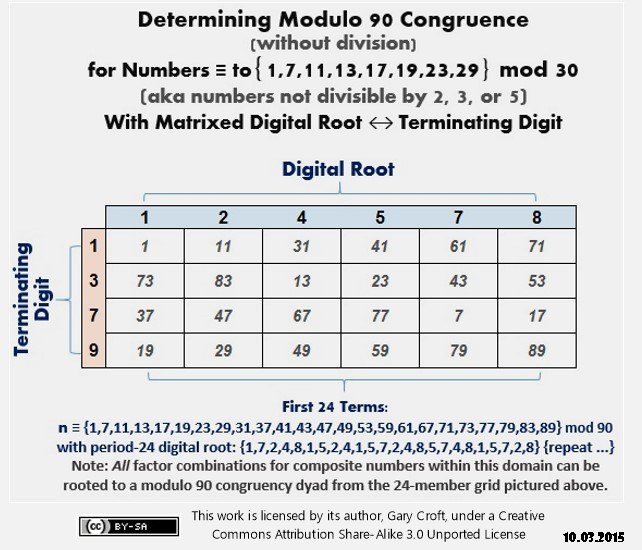### Factorization and Sieving Methods Employing the Prime Spiral Sieve

For a detailed discussion of efficient factorization and prime number sieving algorithms, as well as an in-depth analysis of the 8-chord progression and deterministic modulo 90 digital root dyad sequences underlying all factorizations employing this sieve, click here: Factorization and Prime Number Sieving Algorithms.

If you use Python (the computer programming language designed for the development of scientific, engineering and mathematics applications) and want to cut to the chase, check out the MIT licensed Python module dubbed "pyprimes." It's designed to speed test non-probabilistic prime number sieving algorithms (i.e., deterministic sieves that precisely account for all prime numbers within a given range). These include the Sieve of Eratosthenes, Wheel Factorization and the Prime Spiral Sieve (referred to by its alternative name, "Croft Spiral Sieve" or simply "Croft Spiral"), here or here at code.google.com. The programmer, we're pleased to report, rates the Prime Spiral Sieve "fastest/best" and recommends it as "the preferred way of generating prime numbers" compared to the several sieves tested.

### Counting Primes

The Prime Spiral Sieve can be used to calculate the number of prime numbers within a stated range (from 0 to n, or π(x) using the standard notation) with absolute precision, albeit in several unconventional steps. It does so by employing the four elementary operations of arithmetic: addition, subtraction, multiplication and division, and extracting one square root. You could say that this approach is more recipe than function, algorithm, or asymtotic analysis. Regardless, what makes this method novel is that it parses and gives the number of primes without the need to identify any specific primes other than the first three: 2, 3, and 5, i.e., there's no requirement for primality testing, per se. For an in depth exploration of this methodology, click here: Prime Number Counting Methodology

### Twin Primes

The world of twin primes is truly magical. For a detailed discussion of the factorization algorithms and symmetry groups (permutating matrices, orthogonal Latin squares, palindromes, equilateral triangles, complex polygons, etc.) that ultimately determine the distribution of twin primes along three modulo 30 'channels' go to this page: Twin Primes Demystified: Distribution Algorithms and Symmetries.

There you will be introduced to the beautiful 'Palindromagon' (pictured below), a complex polygon generated by tiered digital root dyad cycles central to the twin prime distribution channels (as well as modulo 90 factorization sequences). Its name comes from the fact that the triangulations generating it sum to a period-18 palindrome consisting of the six possible permutations of {3,6,9}, which in turn can be permutated to produce two 3 x 3 Latin squares with rows, columns and principal diagonals all summing to 18:### Distribution of Perfect Squares

All perfect squares within our domain (numbers not divisible by 2, 3 or 5) possess a digital root of 1, 4 or 7 and are congruent to either {1} or {19} modulo 30. By definition, this includes the squares of all prime numbers greater than 5. We can easily explain this from a digital root perspective given that the digital roots of members of our domain are restricted to 1, 2, 4, 5, 7 or 8 (Numbers with digital root 3, 6, or 9 can't be members because they are divisible by 3.). Thus the digital root of squares is likewise restricted, as follows (and note the palindrome):

1 x 1 = 1
2 x 2 = 4
4 x 4 = 7
5 x 5 = 7
7 x 7 = 4
8 x 8 = 1

By arithmetic law, perfect squares can only have terminating digits of 1, 4, 5, 6, 9 or 0. Only two of these final digits (1 and 9) apply to our domain, i.e., for numbers congruent to {1, 11, 19 or 29} modulo 30. In turn, numbers congruent to {11, 29} sequence digital roots 2, 5 or 8, and therefore – as we demonstrated above – there can be no perfect squares among them. And so it is that the distribution of squares is narrowed to numbers congruent to {1, 19} modulo 30, which is to say they distribute along two – and only two – radii of the Prime Spiral Sieve: 12° (numbers congruent to {1} modulo 30) and 228° (numbers congruent to {19} modulo 30). (This is also consistent with the fact that the quadratic residues for modulo 30 (making them congruent with perfect squares) are 1 and 19.)

[And it follows that all squares in this series distribute evenly to two of the three twin prime distribution channels, described above, negating a significant percentage of potential twin prime pairs.]

The matrix below illustrates the distribution of squares from 1*1 thru 59*59 (squares hi-lited in blue):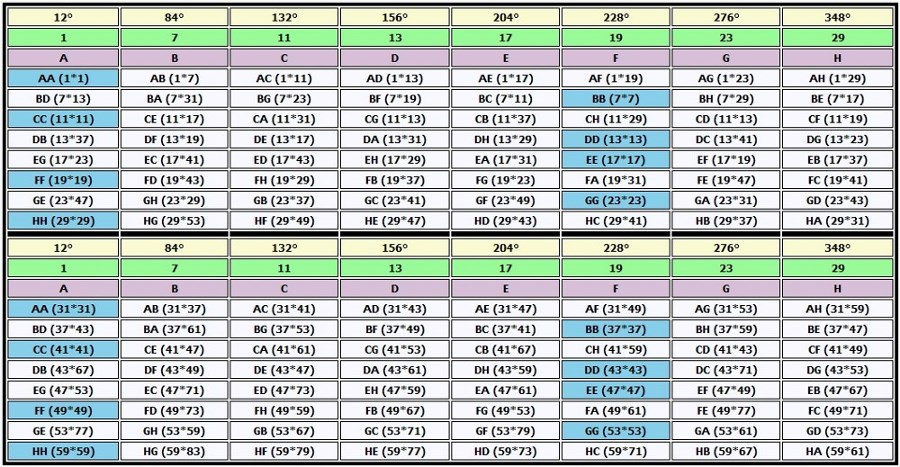Summarizing the above relationships in mathematical terms (and in the knowledge that these modular relationships apply to the squares of all prime numbers ≥ 7) we get:

for all n where n mod 30 = 1, n2 mod 30 = 1
for all n where n mod 30 = 29, n2 mod 30 = 1
for all n where n mod 30 = 11, n2 mod 30 = 1
for all n where n mod 30 = 19, n2 mod 30 = 1

~~~~~~~~~~~~~~~~~~~~~~~~~~~~~~~~~~~~~~~~~~

for all n where n mod 30 = 7, n2 mod 30 = 19
for all n where n mod 30 = 23, n2 mod 30 = 19
for all n where n mod 30 = 13, n2 mod 30 = 19
for all n where n mod 30 = 17, n2 mod 30 = 19

~~~~~~~~~~~~~~~~~~~~~~~~~~~~~~~~~~~~~~~~~~

When the digital root of perfect squares is sequenced within a modulo 30 x 3 = modulo 90 horizon, beautiful symmetries in the form of period-24 palindromes are revealed, which the author has documented on the On-Line Encyclopedia of Integer Sequences as Digital root of squares of numbers not divisible by 2, 3 or 5 (A24092):

1, 4, 4, 7, 1, 1, 7, 4, 7, 1, 7, 4, 4, 7, 1, 7, 4, 7, 1, 1, 7, 4, 4, 1

In the matrix pictured below, we list the first 24 elements of our domain, take their squares, calculate the modulo 90 congruence and digital roots of each square, and display the digital root factorization dyad for each square (and map their collective bilateral 9 sum symmetry):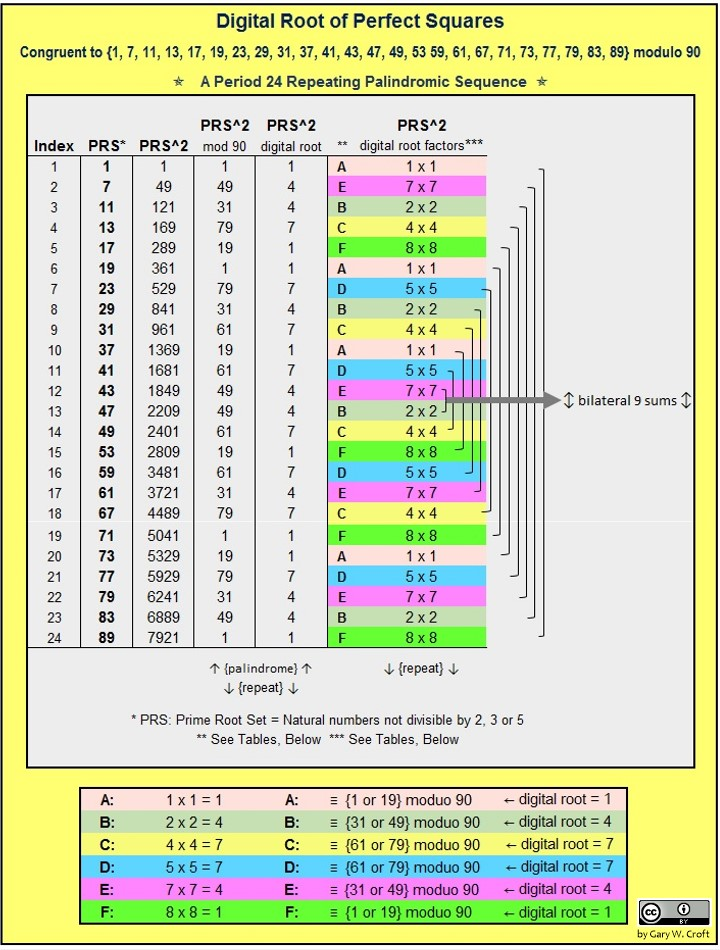Here's one more modulo 90 spin on perfect squares. Parsing the squares by their mod 90 congruence reveals that there are 96 perfect squares generated with each 4 * 90 = 360 degree cycle, which distribute 16 squares to each of 6 mod 90 congruence sub-sets defined as n congruent to {1, 19, 31, 49, 61, 79} forming 4 bilateral 80 sums. Note that each of the 6 columns has 8 bilateral 360 sums, tor a total of 48 * 360 = 40 * 432 (much more on the significance of number 432, elsewhere on this site). Here's the graphic: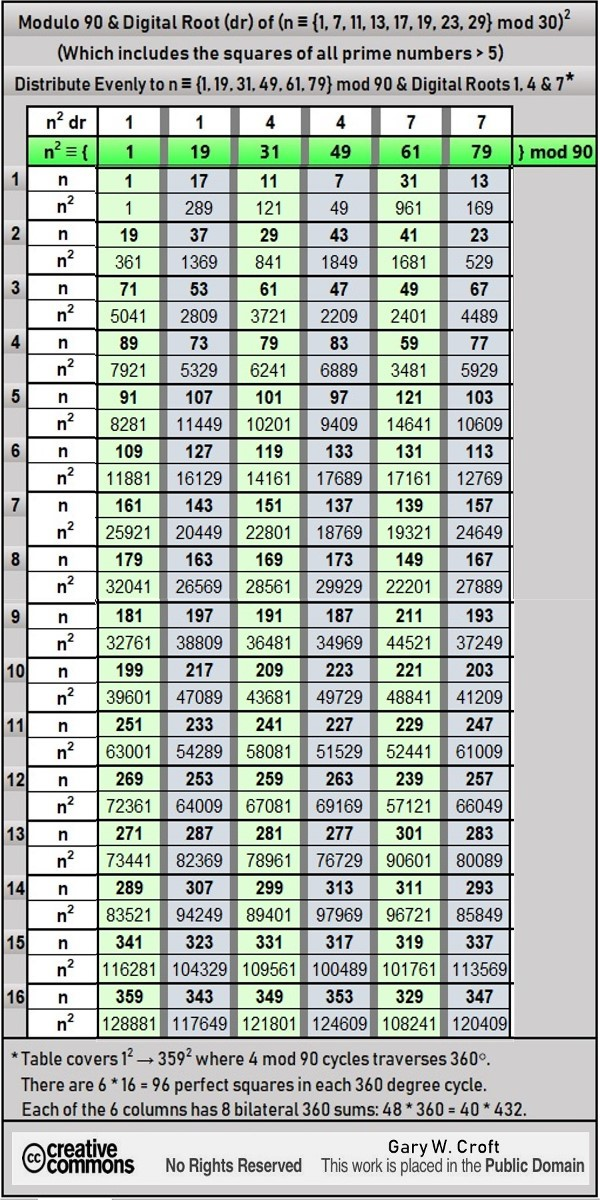Before leaving the subject of perfect squares, there's another hidden dimension of our domain worth noting involving multiples of 360, i.e., when framed as n ≌ {1, 7, 11, 13, 17, 19, 23, 29, 31, 37, 41, 43, 47, 49, 53 59, 61, 67, 71, 73, 77, 79, 83, 89} modulo 90, and taking 'bipolar' differentials of perfect squares, as follows:

(892 − 12) = 360 x 22
(832 − 72) = 360 x 19
(792 − 112) = 360 x 17
(772 − 132) = 360 x 16
(732 − 172) = 360 x 14
(712 − 192) = 360 x 13
(672 − 232) = 360 x 11
(612 − 292) = 360 x 8
(592 − 312) = 360 x 7
(532 − 372) = 360 x 4
(492 − 412) = 360 x 2
(472 − 432) = 360 x 1

### From Alpha to Omega

"Not every beautiful theory has a physical application, but all fundamental physical theories found so far–without exception–have a type of beauty or symmetry." – Michio Kaku, The God Equation

The Ulam Spiral arrays prime numbers in fragmented spiral and diagonal formations. Quoting from Wikipedia: "Since in the Ulam spiral adjacent diagonals are alternatively odd and even numbers, it is no surprise that all prime numbers lie in alternate diagonals ... What is startling is the tendency of prime numbers to lie on some diagonals more than others." From this one might deduce that the Ulam Spiral is very likely a scrambled version of the Prime Spiral Sieve as the latter demonstrates how all prime numbers (except 2, 3 and 5) are fundamentally arrayed along eight (and only eight) diagonals.

It would appear, circumstantially, that the Prime Spiral Sieve is mathematically harmonious and perhaps isomorphic with the most complex and visually arresting Lie group, named E8, which–like the Prime Spiral Sieve–is 8-dimensional (E8 is pictured below superimposed with a star polygon and the 8 radii of the modulo 30 factorization wheel). This group was recently in the news as possibly being a key to unifying theories in gravity and particle physics to create the proverbial "theory of everything." The number 30–integral to the Prime Spiral Sieve–is the Coxeter Group number h, dual Coxeter number and the highest degree of fundamental invariance of E8. You'll note, looking at the graphical representation of E8 below, that the perimeters of every one of its multiple concentric circles possesses 30 points. And, not surprisingly, E8 has 2-, 3- and 5-torsion and its exponents are the co-primes up to 30, i.e., 1, 7, 11, 13, 17, 19, 23, and 29–numbers you're very familiar with if you've read to this point ... which brings us full circle Ο:The Number 30 in Lie Group E8This graphic superimposes images of the star polygon, modulo 30 wheel factorization radii and "E8 graph of the Gosset 421 polytope as a 2-dimensional skew orthogonal projection inside Petrie polygon ... an emulation of the hand drawn original by Peter McMullen" licensed by Creative Commons; license terms here. (For 3-D version of this graphic step back three feet from the screen.)

We'll close with a graphic showing E24 superimposed with the 24 radials of a modulo 90 factorization wheel and the 15 points of a 15-point star represented with red dots, each point separated by 24° (and we see that all nine twin prime distribution channels are hit dead center).